## Wednesday, December 28, 2005

### Math Tricks ... Numeric Vispo?

Vê o que acontece se multiplicarmos 37 por múltiplos de 3:

3 x 37 = 111
6 x 37 = 222
9 x 37 = 333
12 x 37 = 444
15 x 37 = 555
18 x 37 = 666
21 x 37 = 777
24 x 37 = 888
27 x 37 = 999

Agora vê isto:

111111111 x 111111111 = 12345678987654321

Repara neste trapézio:

1 x 9 + 2 = 11
12 x 9 + 3 = 111
123 x 9 + 4 = 1111
1234 x 9 + 5 = 11111
12345 x 9 + 6 = 111111
123456 x 9 + 7 = 1111111
1234567 x 9 + 8 = 11111111
12345678 x 9 + 9 = 111111111

E neste outro:

1 x 8 + 1 = 9
12 x 8 + 2 = 98
123 x 8 + 3 = 987
1234 x 8 + 4 = 9876
12345 x 8 + 5 = 98765
123456 x 8 + 6 = 987654
1234567 x 8 + 7 = 9876543
12345678 x 8 + 8 = 98765432
123456789 x 8 + 9 = 987654321

E ainda neste:

0 x 9 + 8 = 8
9 x 9 + 7 = 88
98 x 9 + 6 = 888
987 x 9 + 5 = 8888
9876 x 9 + 4 = 88888
98765 x 9 + 3 = 888888
987654 x 9 + 2 = 8888888
9876543 x 9 + 1 = 88888888
98765432 x 9 + 0 = 888888888
987654321 x 9 - 1 = 8888888888
9876543210 x 9 - 2 = 88888888888

I find these little pieces very interesting.

These have been floating around on the web lately and were emailed to me twice by different people. I have no idea how old they are or who originally discovered them. I would imagine that more than one person contributed to this group. I would not call them mathematical poetry but they may be considered some form of numeric Vispo.

They remind me a little bit of magic squares.
http://mathforum.org/alejandre/magic.square.html

If odd math expressions are your thing you may want to check the following:
http://curiousmath.com/

Happy Holidays

## Saturday, December 17, 2005

### American Mathematical Society has accepted "Golden Fear"

GOLDEN FEAR
by KAZ MASLANKA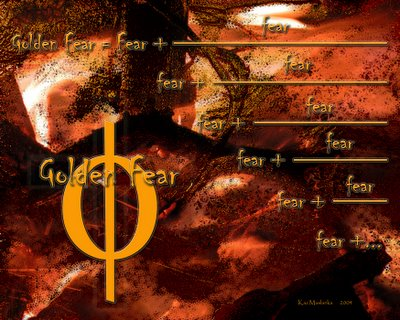The American Mathematical Society has accepted “Golden Fear” in their upcoming show in San Antonio, Texas
Here is a link to the other Mathematical Artworks accepted in the show.

http://myweb.cwpost.liu.edu/aburns/jmm06/jmm06.htm

“Golden Fear” uses one of the equations for the golden ratio or phi, which is the most irrational number compared with all irrational numbers. Out of all the equations for phi “Golden Fear” uses the equation, which is in the form of a continued fraction. However, the word ‘fear’ is substituted for the number '1' in the original equationhttp://mathworld.wolfram.com/GoldenRatio.html

## Monday, December 12, 2005

### Power of Confidence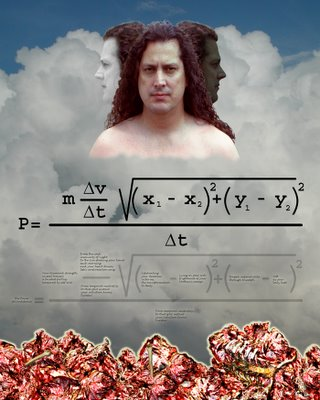Bob Grumman reviewed a couple of pieces of mine on his blog and I would like to share my comments to him.
http://comprepoetica.com/newblog/blog00676.html

Bob,
I appreciate the posting and would like to comment.

Bob said,” To be frank, I'm not sure why he seems to provides a range of values for his delta time,”

Delta means the “change in” see the link below for they explain it quite well. In a nutshell, when delta is present one must put things in terms of what it changes to from what state it originally had. So it goes that the change in x would be between two different states of x. In equation form it follows that (x1-x2) or basically the change from the existing state of x1 to the change of the new state, which is x2. That is why we had two different states in the poem … one being temporal neutrality and the other being the state of eureka

what are deltas?

Concerning the relationship of the Images to the math, I view the relationship of the images to the math poem the same as one would view the music in relation to the lyrics of a song … As to the task of putting my work in terms of Vispo … I guess my work would fit under the category of emblem poems with math poems instead ‘text only’.

Your Blog entry has inspired me to go into detail about how this piece works. The physics equation for Power states that power is equal to energy expended per duration of time. That’s all there is to it … That is the definition of power. (The term work and energy are synonymous)

Understanding the equation for power

However, there is much to what energy means. So now let us look at what physics says about energy.

Different faces of energy

In my piece Energy is the amount of force exerted over a distance. Let’s talk about distance. Distance is basically the Pythagorean Theorem mapped out on a Cartesian coordinate system. What we have is a change in position in one axis relative to a change in position in another perpendicular axis. (The following link explains it quite well)

http://www.purplemath.com/modules/distform.htm

Because there is a relationship between Energy and force lets look at what physics says about force. Force is mass multiplied by acceleration and acceleration is the change in velocity per time. (Delta t) So lets put the puzzle altogether and see how it reads.(in two dimensions) Power is a mass multiplied by a change in velocity per time multiplied by a change in position in one axis relative to a change in position in another axis and all of the previous verbiage is divided by a change between two positions in time.

Now let’s put the equation for power in context with my piece “The power of confidence”

Let me introduce a term … “virtual object” can be an emotional or psychological object present in my mathematical poetry that utilizes physics equations as a paradigm “to carry” the poem. The virtual object is a substitute for the physical object that is normally the subject of a physics equation.

The mass is of our virtual object is “Your diamond strength crystal bravery, a faceted fortress tempered by your self love”

The change in velocity 'delta v' of our virtual object = “From the cold insecurity of night to the sun shaking your hand each morning while your heart drums life's confirmation song”
The change in time that affects the virtual object 'delta t' = “From temporal neutrality to that split instant your intuition knows . . . eureka!”

The distance our virtual object will traverse:
The difference between x1 and x2 in our first dimension:

x1 = “Celebrating your demonic victories, the transformation to deity”

Furthermore, the difference between y1 and y2 in our second dimension:

y1 = “Forged impenetrable through triumph”
y2 = “Soft as your baby butt”

The Poem in words:
So you can see that our mass of “Your diamond strength crystal bravery, a faceted fortress tempered by your self love” is being accelerated between the concepts of “the cold insecurity of night” to the concept of “the sun shaking your hand each morning while your heart drums life's confirmation song” and this is happening between the moments of “temporal neutrality” to the moment of “that split instant your intuition knows . . . eureka!” and all of this is moving over the distance between the difference of the concepts of “Celebrating your demonic victories, the transformation to deity” and “Lying in your crib, frightened of your mothers sneeze” and moving in a second dimension over the distance between the difference of the concepts of “Forged impenetrable through triumph” and “Soft as your baby butt” Furthermore all of this is occurring between the moments of “temporal neutrality” to the moment of “ that split instant your intuition knows . . . eureka!”

## Thursday, December 08, 2005

### Beginner's Mind ~ Denotation / Connotation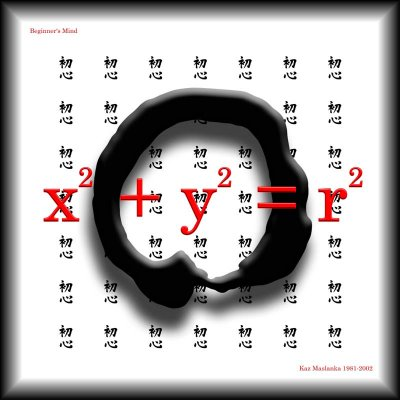Here are two forms of perfection in a artwork entitled “beginners mind”. The first being the Buddhist Calligraphic circle called Enso in Japan and Ilwonsang in Korea. A perfect example of circular perfection expressed as connotation … and printed on top of that, we have the mathematical equation for a circle: x^2 + y^2 = r^2 … which is a perfect example of circular perfection expressed as denotation.

http://www.byzant.com/symbols/enso.asp
link to equation of a circle: (my example h and k are valued at zero)
http://www.analyzemath.com/CircleEq/Tutorials.html

A moment of silence for John Lennon ...

## Monday, December 05, 2005

### AMS American Mathematical Society Conference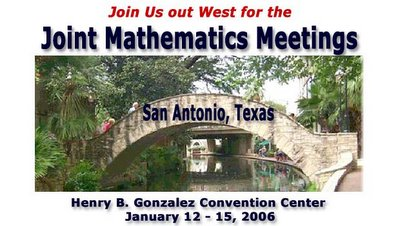I plan on attending this conference ... It should be a good time.

check out their web site.

http://www.ams.org/amsmtgs/2095_exhibits.html

## Sunday, December 04, 2005

### Mathematical Simile Mathematical Metaphor

Mathematical simile is the function of virtually all applied math equations for which the public is familiar (usually scientific). For example physics equations are paradigms used to correlate experience. Mathematical models can be made for anything rationally understood and are descriptive of the relationships between the elements described.
A simile is a paradigm. One can see this expressed in the physics equation distance is equal to velocity multiplied by time. When you apply this equation to a situation you realize that this is an approximation even for all practical purposes it accomplishes the task at hand. The bottom line is that it is an approximation even though the equation expresses it as exact. The math equation is exact the application is not. Therefore it is simile ‘our experience of distance is like velocity multiplied by time.’ One may argue that the physics equation is metaphor precisely for the same reason e.g. we are saying that distance is exactly equal to velocity multiplied by time and it is not. Therefore we have a logical tension that creates the metaphor. The next step is to look at the intention of the equation, whether it is intended for connotation or denotation. Clearly physics equations are intended for denotation. Therefore what is really important for us is the intention of the equation. This also illuminates the idea that there are a lot of shades of gray between simile and metaphor yet we don’t usually think in this manner. I know a lot of people that think simile and metaphor are really the same. I believe at some point there is a nonsense threshold that one crosses and you can take it no other way except metaphor. Unfortunately that threshold line can be anywhere on a number line between the concept of simile and metaphor. Personally I see that threshold very close to simile. That is as soon as it starts getting nonsensical I consider it metaphor.

The visual image presented takes the following simile and expresses it as metaphor.

Although it may or may not be universally true I find that a man’s intelligence is inversely proportional to the levels of alcohol and testosterone in his system. I gave a lecture last week to a group of art students from Santa Ana Art Institute (Thank you Professor Ben Miles) and when I proposed this statement I got a bunch of laughs and a lot of nodding heads. Consequentially I believe there is some truth in there.## Friday, December 02, 2005

### Humor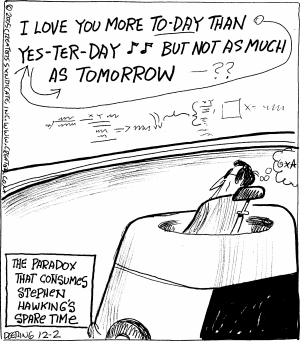Tim Repp sent me this little mathpo related humor

## Thursday, December 01, 2005

### Synecdochical Physics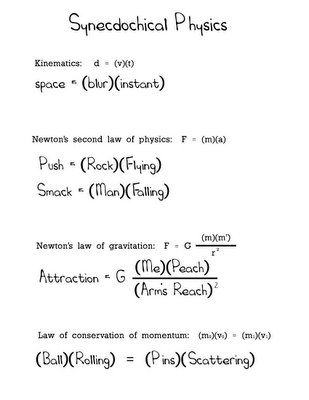I was inspired by conversations with The Mathematician Paul Gailiunas and a blog entry of Visual Poet Geof Huth to produce some synecdoche physics equations.

syn·ec·do·che n. Listen:
A figure of speech in which a part is used for the whole (as hand for sailor), the whole for a part (as the law for police officer), the specific for the general (as cutthroat for assassin), the general for the specific (as thief for pickpocket), or the material for the thing made from it (as steel for sword).

Whereas in all of the equations the variables, for the physics equations, have been replaced with a synecdoche counter part not all of the equations as a whole could be considered synecdochical. All of them but one created a synecdochical equation.

Newton’s equation on gravity is actually a metaphor. That is due to the fact that the force variable (replaced by attraction) in the mathpoem does not pertain to gravity but is in reference to the attraction caused by hunger. If it were pertaining to gravity then the equation would be synecdochical however anything else makes the equation a metaphor.

http://dbqp.blogspot.com/2005/10/synechdoche-explained.html